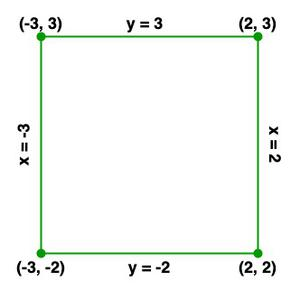Open in App
Not now

# Class 11 RD Sharma Solutions- Chapter 23 The Straight Lines- Exercise 23.2

• Difficulty Level : Hard
• Last Updated : 19 Jan, 2021

### Question 1. Find the equation of the line parallel to the x-axis and passing through (3, -5).

Solution:

Let the equation of line be:

y – y1 = m(x – x1)

As line is parallel to x-axis, so slope(m) of the line would be equal to 0 (i.e. m=0).

Here (x1, y1) = (3, -5)

So,

y – (-5) = 0 (x – 3)

y + 5 = 0

So, the required equation of the line is y + 5 = 0 or y = -5.

### Question 2. Find the equation of the line perpendicular to the x-axis and having intercept -2 on x-axis.

Solution:

Any line perpendicular to x-axis will have slope = -1/0

Let the equation of line be:

y – y1 = m(x – x1)

Here m = -1/0 and (x1, y1)= (-2, 0)

y – 0 = (-1/0)(x – (-2))

y – 0 = (-1/0)(x + 2)

-(x + 2) = 0

x + 2 = 0

So the required equation of the line is x + 2 = 0 or x = -2.

### Question 3. Find the equation of the line parallel to the x-axis and having intercept -2 on the y-axis.

Solution:

The slope of x-axis is 0 and line parallel to x-axis will also have same slope, therefore m = 0

Line has intercept -2 on y-axis

Therefore, (x1, y1) = (0, -2)

Let the equation of line be :

y – y1 = m(x – x1)

y – (-2) = 0(x – 0)

y + 2 = 0

So, the required equation of the line is y + 2 = 0 or y = -2.

### Question 4. Draw the line x = -3, x = 2, y = -2, y = 3, and write the coordinates of the vertices of the square so formed.

Solution:

The figure with the lines x = -3, x = 2, y = -2, y = 3 is as follows:From the above figure, we can say that the coordinates of the vertices of the square are:

(2, 3), (-3, 3), (-3, -2), (2, -2).

### Question 5. Find the equations of the straight lines which pass through (4, 3) and are respectively parallel and perpendicular to the x-axis.

Solution:

Slope of line parallel to x-axis = 0

As the line passes through (4, -3)

The required equation of the line parallel to x-axis is

y – y1 = m(x – x1)

y – (3) = 0(x – 4)

y – 3 = 0

y = 3

Slope of a line perpendicular to x-axis = -1/0

The required equation of the line perpendicular to x-axis is

y – y1 = m(x – x1)

y – 3 = (-1/0)(x – 4)

x – 4 = 0

x = 4

### Question 6. Find the equation of a line which is equidistant from the lines x = -2 and x = 6.

Solution:

The lines x = -2 and x = 6 pass through the points (-2, 0) and (6, 0) respectively.

Let (h, k) be the mid-point of the line joining the points (-2, 0) and (6, 0).

Therefore, (h, k)=((-2 + 6) / 2, 0) = (2, 0)

The given lines are parallel to the y-axis and the required line is equidistant from

these lines. Hence, the required line is parallel to the y-axis, which is given by x = k.

This line passes through (2, 0)

Therefore, 2 – k = 0

k = 2

So, the equation of a line that is equidistant from the lines x = -2 and x = 6 is x = 2.

### Question 7. Find the equation of a line equidistant from the lines y = 10 and y = -2.

Solution:

The lines y = 10 and y = -2 pass through the points (0, 10) and (0, -2) respectively.

Let (h, k) be the mid-point of the line joining the points (0, 10) and (0, -2).

Therefore, (h, k) = (0, (10 – 2) / 2) = (0, 4)

The given lines are parallel to the x-axis and the required line is equidistant from

these lines. Hence, the required line is parallel to the y-axis, which is given by y = k.

This line passes through (0, 4)

Therefore, k = 4

So, the equation of a line that is equidistant from the lines y = 10 and y = -2 is y = 4.

My Personal Notes arrow_drop_up